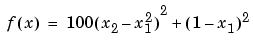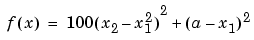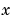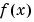MATLAB Function Referencefminsearch

Minimize a function of several variables

Syntax

• ```x = fminsearch(fun,x0)
x = fminsearch(fun,x0,options)
[x,fval] = fminsearch(...)
[x,fval,exitflag] = fminsearch(...)
[x,fval,exitflag,output] = fminsearch(...)
```

Description

fminsearch finds the minimum of a scalar function of several variables, starting at an initial estimate. This is generally referred to as unconstrained nonlinear optimization.

```x = fminsearch(fun,x0) ``` starts at the point `x0` and finds a local minimum `x` of the function described in `fun`. `x0` can be a scalar, vector, or matrix. `fun` is a function handle. See Function Handles in the MATLAB Programming documentation for more information.

Parameterizing Functions Called by Function Functions, in the MATLAB mathematics documentation, explains how to provide additional parameters to the function `fun`, if necessary.

```x = fminsearch(fun,x0,options) ``` minimizes with the optimization parameters specified in the structure `options`. You can define these parameters using the `optimset` function. `fminsearch` uses these `options` structure fields:

 `Display` Level of display. `'off'` displays no output; `'iter'` displays output at each iteration; `'final'` displays just the final output; `'notify'` (default) displays output only if the function does not converge. `FunValCheck` Check whether objective function values are valid. `'on'` displays a warning when the objective function returns a value that is `complex`, `Inf` or `NaN`. `'off'` (the default) displays no warning. `MaxFunEvals` Maximum number of function evaluations allowed `MaxIter` Maximum number of iterations allowed `OutputFcn` Specify a user-defined function that the optimization function calls at each iteration. `TolFun` Termination tolerance on the function value `TolX` Termination tolerance on `x`

```[x,fval] = fminsearch(...) ``` returns in `fval` the value of the objective function `fun` at the solution `x`.

```[x,fval,exitflag] = fminsearch(...) ``` returns a value `exitflag` that describes the exit condition of fminsearch:

 `1` `fminsearch` converged to a solution `x`. ` 0` Maximum number of function evaluations or iterations was reached. `-1` Algorithm was terminated by the output function.

```[x,fval,exitflag,output] = fminsearch(...) ``` returns a structure `output` that contains information about the optimization:

 `output.algorithm` Algorithm used `output.funcCount` Number of function evaluations `output.iterations` Number of iterations `output.message` Exit message

Arguments

`fun` is the function to be minimized. It accepts an input `x` and returns a scalar `f`, the objective function evaluated at `x`. The function `fun` can be specified as a function handle for an M-file function

• ```x = fminsearch(@myfun, x0)
```

where `myfun` is an M-file function such as

• ```function f = myfun(x)
f = ...            % Compute function value at x
```

or as a function handle for an anonymous function, such as

• ```x = fminsearch(@(x)sin(x^2), x0);
```

Other arguments are described in the syntax descriptions above.

Examples

Example 1. A classic test example for multidimensional minimization is the Rosenbrock banana function

•The minimum is at `(1,1)` and has the value `0`. The traditional starting point is `(-1.2,1)`. The anonymous function shown here defines the function and returns a function handle called `banana`:

• ```banana = @(x)100*(x(2)-x(1)^2)^2+(1-x(1))^2;
```

Pass the function handle to `fminsearch`:

• ```[x,fval] = fminsearch(banana,[-1.2, 1])
```

This produces

• ```x =

1.0000    1.0000

fval =

8.1777e-010
```

This indicates that the minimizer was found to at least four decimal places with a value near zero.

Example 2. If `fun` is parameterized, you can use anonymous functions to capture the problem-dependent parameters. For example, suppose you want to minimize the objective function `myfun` defined by the following M-file function.

• ```function f = myfun(x,a)
f = x(1)^2 + a*x(2)^2;
```

Note that `myfun` has an extra parameter `a`, so you cannot pass it directly to `fminsearch`. To optimize for a specific value of `a`, such as `a = 1.5`.

1. Assign the value to `a`.
• ```a = 1.5; % define parameter first
```
2. Call `fminsearch` with a one-argument anonymous function that captures that value of `a` and calls `myfun` with two arguments:
• ```x = fminsearch(@(x) myfun(x,a),0,1)
```

Example 3. You can modify the first example by adding a parameter a to the second term of the banana function:

•This changes the location of the minimum to the point `[a,a^2]`. To minimize this function for a specific value of `a`, for example a = `sqrt(2)`, create a one-argument anonymous function that captures the value of `a`.

• ```a = sqrt(2);
banana = @(x)100*(x(2)-x(1)^2)^2+(a-x(1))^2;
```

Then the statement

• ```[x,fval] = fminsearch(banana, [-1.2, 1], ...
optimset('TolX',1e-8));
```

seeks the minimum `[sqrt(2), 2]` to an accuracy higher than the default on `x`.

Algorithm

fminsearch uses the simplex search method of . This is a direct search method that does not use numerical or analytic gradients.

If `n` is the length of `x`, a simplex in `n`-dimensional space is characterized by the `n+1` distinct vectors that are its vertices. In two-space, a simplex is a triangle; in three-space, it is a pyramid. At each step of the search, a new point in or near the current simplex is generated. The function value at the new point is compared with the function's values at the vertices of the simplex and, usually, one of the vertices is replaced by the new point, giving a new simplex. This step is repeated until the diameter of the simplex is less than the specified tolerance.

Limitations

fminsearch can often handle discontinuity, particularly if it does not occur near the solution. fminsearch may only give local solutions.

fminsearch only minimizes over the real numbers, that is,must only consist of real numbers andmust only return real numbers. Whenhas complex variables, they must be split into real and imaginary parts.

See Also

`fminbnd`, `optimset`, `function_handle` (@), anonymous functions

References

  Lagarias, J.C., J. A. Reeds, M. H. Wright, and P. E. Wright, "Convergence Properties of the Nelder-Mead Simplex Method in Low Dimensions," SIAM Journal of Optimization, Vol. 9 Number 1, pp. 112-147, 1998.

© 1994-2005 The MathWorks, Inc.